### Mathematical Preliminaries: Types of Maps

#### Learning Outcomes:

• Differentiate between injective, surjective, and bijective maps

### Types of Maps

Maps or functions between sets can be injective, surjective, bijective, or none of the above.

#### Injective Maps

An injective map also called “one-to-one” is a map that designates a unique image for every element. Let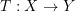.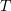is injective if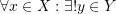such that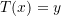.

#### Surjective Maps

A surjective map also called “onto” is a map such that every element in the codomain has a pre-image. Let.is surjective if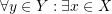such that. In other words, there is always a pre-image for all the elements in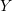.

#### Bijective Maps

A bijective map also called “invertible” is a map such which is both injective and surjective. An bijective maps is a map that can be inverted, i.e., let.is bijective, then, there exists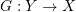such that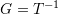. I.e.,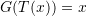.

#### Examples

For example, let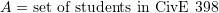and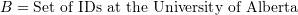. The map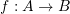assigns every student a unique ID in the set of IDs of students at the University of Alberta. This map is injective or one-to-one since each student has his/her own unique ID. However, this map is not surjective (onto) because there are IDs in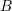that are not assigned to students in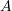. Similarly, letand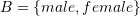. There is a surjective map between the students in the class and the gender types as there are both male and female students taking CivE 398. Unless there is only one male student and one female student, this map is surjective but not injective. Figure 1 contains some illustrative examples of the above definitions.

### Quiz 2 – Types of Maps

#### Solution Guide

1.Qisi says:

Hi there, thank you very much for putting up this book. I’m a bit confused about the definition of injection in the text. For the case of f:R->R:f(x) = x^2, for any x in R, there is indeed a unique y s.t y=x^2. I’m having the feeling that the definition of injection should be something like:
any y in Y s.t. y=f(x), there exists a unique x in X that satisfies y=f(x),
or:
any x1,x2 in X, x1~=x2 => f(x1) ~= f(x2)

2.Samer Adeeb says:

Hi Qisi,
The following two statements are equivalent:
– for all x1~=x2 => f(x1)~=f(x2)
– for all x => f(x) is unique.

3.audeser says:

Under “SURJECTIVE MAPS”, the “Let t : X\rightarrow Y” should be Let T : X\rightarrow Y”

1.Samer Adeeb says:

This has been correct. Thank you.